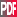18th Conference on Climate Variability and Change

P3.4

Equilibrium Phases in a energy-relative enstrophy Statistical Mechanics model of barotropic flows on a rotating sphere—non-conservation of angular momentum

Xueru Ding, Rensselaer Polytechnic Institute, Troy, NY; and C. Lim

The statistical equilibrium of barotropic flows on a rotating sphere is simulated in a wide range of parameter space by Monte-Carlo methods. A spin lattice Hamiltonian with a canonical constraint on the kinetic energy and a microcanonical constraint on the relative enstrophy is formulated as a convergent family of finite dimensional approximations of the barotropic vortex statistic on the rotating sphere. As this Spin-lattice Hamiltonian model cannot be solved exactly since the nonzero rotation of the sphere will result in a difficult external field like term which is spatially inhomogeneous, Monte-Carlo simulations are used to calculate the spin-spin correlations and the mean nearest neighbor parity as order parameters or indicators of phase transitions in the system. Interestingly we find that at extremely high energy levels or negative temperatures, the preferred state is a superrotational equilibrium state aligned with the planetary spin. For large values of the planetary spin compared with the relative enstrophy there appears to be two phase transitions when the temperture varies from a numerically large negative value to a large positive value. The latter arises as constrained energy minimizers which can undergo a tilt instability of the axis of atmospheric rotation. This means the axis of atmospheric rotation does not always point in exactly the same direction of the axis of planetary spin but wobbles a little. A simple mean field theory which constrains the relative enstrophy only in an averaged sense and relaxes the angular momentum, gives critical temperatures that are consistent with the Monte-Carlo simulations.Extended Abstract (448K)